Describing motion along a line

Start this free course now. Just create an account and sign in. Enrol and complete the course for a free statement of participation or digital badge if available.

Free course

# 3.2 Constant velocity and the gradient of the position-time graph

Two things you will almost certainly want to know about any particle undergoing uniform motion are 'how fast is it travelling?' and 'in which direction is it moving?' The physical quantity that provides both these items of information is the particle's velocity. This is defined as the rate of change of the particle's position with respect to time, and has a constant value for each case of uniform motion along a line.

velocity = rate of change of position with respect to time.

In the case of the car whose position-time graph is shown in Figure 12b, if we choose two different times, say, t﻿1 = 40 s and t﻿2 = 50 s, then in the time interval between t﻿1 and t﻿2, the car moves from x﻿1 = 150 m to x﻿2 = 180 m. It follows that the rate of change of position of the car is given by the ratio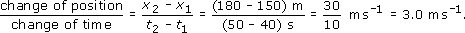We therefore say that the velocity of the car is 3.0 metres per second along the x-axis, which we write using the abbreviation m s−1.

More generally, if any particle moves uniformly along the x-axis, so that its position-time graph is a straight line, then the constant velocity v x (' v sub x') of that particle is given by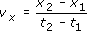where x﻿1 is the particle's position at time t1, and x﻿2 is its position at time t﻿2. Note that the velocity may be positive or negative, depending on whether the particle's position coordinate is increasing or decreasing with time. Also note that for uniform motion the velocity is independent of the particular values of t﻿1 and t﻿2 that are chosen.

## Question 8

Determine the velocity of the car by making measurements on the graph in Figure 12b in the interval from 50 s to 60 s.

Usingand Figure 12b we obtain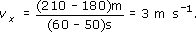A point to note here is that choosing such closely separated values as t = 50 s and t = 60 s makes the evaluation of the velocity trickier, and more prone to error than would have been the case if more widely separated values had been chosen. When evaluating a gradient from a graph, it is always wise to use the widest convenient range of values on the horizontal axis.

In everyday speech the terms velocity and speed are used interchangeably. However, in physics, the term speed is reserved for the magnitude of the velocity, i.e. its value neglecting any overall minus sign. So, if a particle has velocity v﻿﻿﻿x﻿ = −﻿5 m s﻿−1, then its speed is v﻿ = 5 m s﻿−﻿1, where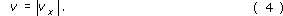Speed is a positive quantity telling us how rapidly the particle is moving but revealing nothing about its direction of motion.

The subscript x in v﻿﻿﻿x may seem a bit cumbersome, but it is an essential part of the notation. The subscript reminds us that we are dealing with motion along the x-axis. In later work, we will need to use two or sometimes three axes, so by including the subscript at this stage, we will be able to use the same notation throughout. Be careful to include the subscript x in your written work and make sure that it is small enough and low enough to be read as a subscript - don't risk having v﻿﻿﻿x﻿ misinterpreted as vx, i.e. v times x.

Another piece of shorthand that you will find useful concerns the upper case Greek letter delta, Δ. If a quantity such as x changes its value from x1 to x2, then the change in the value of x is conventionally written as Δx, and read as 'delta ex'. Thus

Δx = x2x1.

So, Δx and Δt mean the changes in x and t; they do not mean Δ times x or Δ times t. You should always think of the Δ and the symbol that follows it as a single entity; the Δ symbol by itself has no quantitative meaning. Using this notation, the expression for v﻿﻿x above can be written as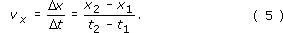In graphical terms, the velocity Δ﻿x﻿/﻿Δ﻿t of a uniformly moving particle is indicated by the slope of its position-time graph. The steepness of the line represents the speed of the particle, while the orientation of the line - bottom left to top right, or top left to bottom right - indicates the direction of motion. If the graph of x against t is a straight line, then the ratio of the change in x to the corresponding change in t (that is Δ﻿x﻿/﻿Δ﻿t﻿) is called the gradient of the graph. A line that slopes from bottom left to top right indicates that a positive change in t corresponds to a positive change in x, consequently such a line has positive gradient. Similarly, a line sloping from top left to bottom right indicates that a positive change in t corresponds to a negative change in x and consequently to a negative gradient. This gives us another way of describing velocity:

the velocity of a particle = the gradient of its position-time graph

## Question 9

Figure 14 shows the position-time graphs for four different objects (A, B, C and D) each moving uniformly with a different constant velocity. The position and time scales are the same in each case.

(a) List the objects in order of increasing speed.

(b) Which of the objects have positive velocity?

(c) List the objects in order of increasing velocity.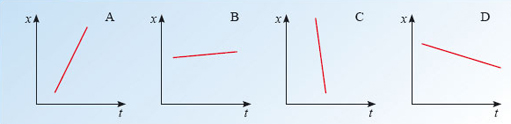Figure 14: Straight line position-time graphs for Self-assessment question 9

(a) The speed is given by the magnitude of Δxt obtained from the velocity-time graph. In the order of increasing speed, the objects are B, D, A, C.

(b) The objects with positive velocity have positive values of Δxt, these are A and B.

(c) Treating large negative values as being less than small negative values, and any negative value as less than any positive value, as is conventional, the list is C, D, B, A.

The gradient of a graph is one of the most important concepts of this course. It is important that you should be able to evaluate gradients, and that you should be able to distinguish graphs with positive gradients from those with negative gradients. It is also important that you should be able to interpret gradients physically as rates of change. In this discussion of position-time graphs the gradient represents velocity, but in other contexts, where quantities other than x and t are plotted, the gradient may have a very different interpretation. Figure 15, for example, shows how the average temperature T of the atmosphere depends on the height h above sea-level. The gradient of this graph, Δ﻿T﻿/﻿Δh, describes the rate at which temperature changes with height.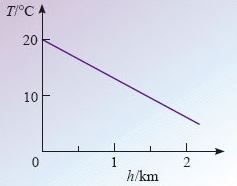Figure 15: A graph of average atmospheric temperature T against height h above sea-level

## Question 10

Estimate the gradient of the graph in Figure 15. (The use of the word 'estimate' implies that you do not need to take great care over the values you read from the graph, but you should take care over matters such as signs and units of measurement.)

The gradient of the temperature-height graph is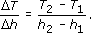With h1 = 0 and h2 = 2 km, Figure 15 shows that T1 = 20 °C and T2 = 6 °C.

It follows that the gradient is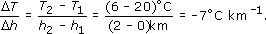Note that care has to be taken to arrange the values in the correct order when performing the subtractions if the right sign (minus in this case) is to be obtained.

S207_2